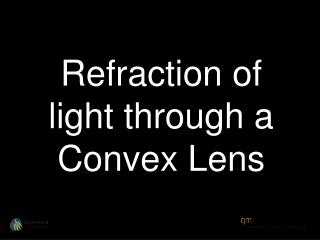DownloadDownload PresentationRefraction of light through a Convex Lens

# Refraction of light through a Convex Lens

Télécharger la présentation## Refraction of light through a Convex Lens

- - - - - - - - - - - - - - - - - - - - - - - - - - - E N D - - - - - - - - - - - - - - - - - - - - - - - - - - -
##### Presentation Transcript

1. Refraction of light through a Convex Lens

2. Lens diagram a cross section through the centre plane.

3. Simple convex (curves outwards) lens

4. Simple concave (curves inwards) lens

5. Convex lens Concave lens

6. Air Lens Air

7. Light ray

8. Light ray is horizontal (at a right angle) to vertical lens

9. Light ray is horizontal (at a right angle) to vertical lens

10. Light ray is horizontal (at a right angle) to vertical lens Extension of right ray

11. Light ray is horizontal (at a right angle) to vertical lens Extension of right ray

12. Light ray is horizontal (at a right angle) to vertical lens Extension of right ray Centre line of the lens

13. Light ray is horizontal (at a right angle) to vertical lens Extension of right ray Centre line of the lens

14. Light ray is horizontal (at a right angle) to vertical lens Extension of right ray Right angle to the centre line of the lens Centre line of the lens

15. The perpendicular

16. The perpendicular

17. The perpendicular Right angle to the surface of the lens